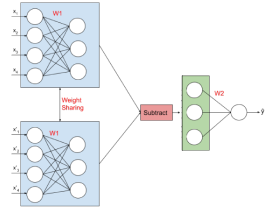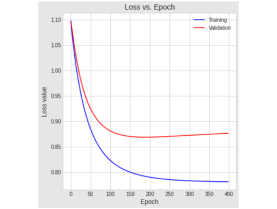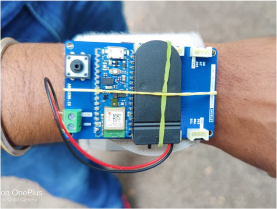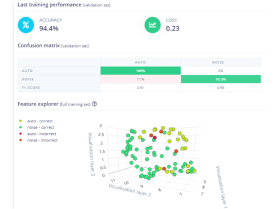## Deep Learning Principles & Applications

Deep learning is a widely and immediately applicable AI tool that is actively used in several disciplines across engineering, natural sciences, health care, finance, and humanities. This workshop aims to provide the participants a hands-on computation-based introduction to the principles and applications of deep learning. Leveraging on a quick, yet accessible introduction to key ideas from linear algebra, participants will be introduced to building a simple neural network from scratch and understanding the fundamental operating principle—the “learning” process. Seamless extension to deep neural networks will follow with multidisciplinary computational examples.

## Course Content

• Essential linear algebra for deep learning
• Fundamentals of linear classification: weights, bias, scores, and loss functions
• Calculus for the gradient descent algorithm
• Forward and backward propagation with regularization
• Batch processing for large datasets
• Linear to nonlinear classification via activation functions
• Computational setup of a shallow neural network
• Tuning neural network performance
• Pre-processing data and batch normalization
• Cross-validation for validating model performance
• Extending the computational setup from a shallow to a deep neural network
• Introduction to the TensorFlow library
• Application projects: implementing shallow and deep neural network models using TensorFlow; implementing machine learning models on edge devices using Edge Impulse.